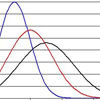#### You may also like### Over-booking

The probability that a passenger books a flight and does not turn up is 0.05. For an aeroplane with 400 seats how many tickets can be sold so that only 1% of flights are over-booked?### Into the Normal Distribution

Investigate the normal distribution### Normal Intersection

Explore the intersection possibilities for normal pdfs.

# Aim High

##### Age 16 to 18Challenge Level

A farmer has a very large farm which produces wheat. The yield of wheat per hectare is known to be normally distributed at $7.74$ tonnes per hectare, with a standard deviation of $0.62$.

Why can a normal distribution of a yield never be entirely accurate? Why, in this case, does it not matter?

It is nearly planting time and the farmer has future orders for $8000$ tonnes of wheat. If he fails to produce enough wheat then he will have to pay a stiff penalty to the buyer; if he produces too much wheat then he will have to offload or destroy the surplus at a loss. In each case, the loss $L$ can be modelled as follows, where $A$ is the amount he plants:

\begin{align*} &\mbox{If } A < 8000, L = (8000 - A)\times 4\\ &\mbox{If } A > 8000, L = 0.5 \times (A-8000)\\\end{align*}

There are three possible levels of analysis of this problem

Level 1 - using confidence intervals
How much wheat would the farmer have to plant to have an expected yield of exactly $8000$ tonnes? What distribution would the total yield have, and what would be the $95\%$ confidence interval for the actual yield?

In this case, what would be the $95\%$ confidence interval for the loss?

How would this alter if he instead planted $1000$ hectares? Could you recommend an ideal amount of wheat to plant?

Level 2 - finding an expected loss
For your planting recommendation, what would be the expected loss?

Level 3 - minimising the expected loss (involves difficult, but fun, calculus)
How much should the farmer plant to minimise his expected loss? Make an initial considered estimate before performing a calculation.